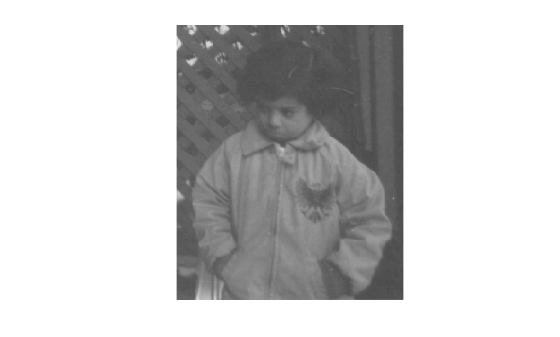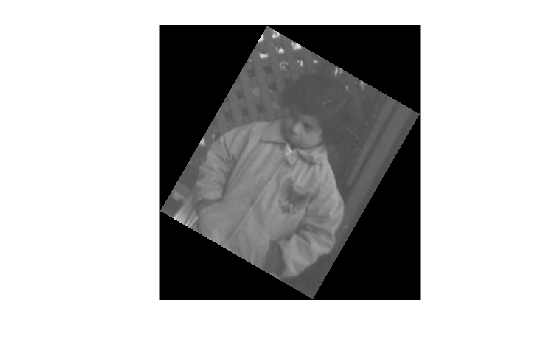Main Content

# invert

Invert geometric transformation

## Syntax

``invtform = invert(tform)``

## Description

example

````invtform = invert(tform)` returns the inverse of the geometric transformation `tform`.```

## Examples

collapse all

Read and display an image.

```I = imread('pout.tif'); imshow(I)```Create an `affine2d` object that defines a 30 degree clockwise rotation around the origin. View the transformation matrix stored in the `T` property.

```theta = 30; tform = affine2d([cosd(theta) sind(theta) 0; -sind(theta) cosd(theta) 0; 0 0 1]); tform.T```
```ans = 3×3 0.8660 0.5000 0 -0.5000 0.8660 0 0 0 1.0000 ```

Apply the forward geometric transformation, `tform`, to the image. Display the rotated image.

```J = imwarp(I,tform); imshow(J)```Invert the geometric transformation. The result is a new `affine2d` object that represents a 30 degree rotation in the counterclockwise direction.

```invtform = invert(tform); invtform.T```
```ans = 3×3 0.8660 -0.5000 0 0.5000 0.8660 0 0 0 1.0000 ```

Apply the inverse geometric transformation, `invtform`, to the rotated image `J`. The final image, `K`, has the same size, shape, and orientation as the original image. Black padding around the image results from the two transformations.

```K = imwarp(J,invtform); imshow(K)```## Input Arguments

collapse all

Geometric transformation, specified as an `affine2d`, `affine3d`, `rigid2d`, `rigid3d`, or `projective2d` geometric transformation object.

## Output Arguments

collapse all

Inverse geometric transformation, returned as a geometric transformation object. `invtform` is the same type of object as `tform`.

## See Also

Introduced in R2013a

Download ebook